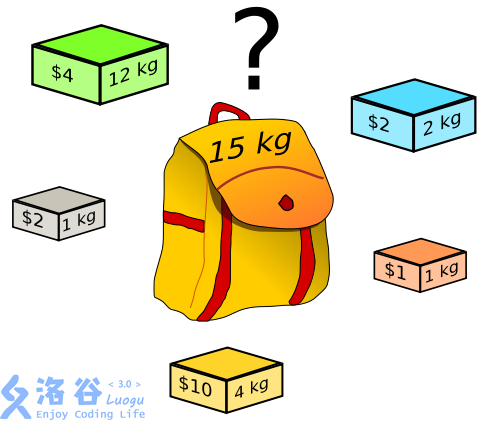# 洛谷P4141消失之物

## 题目描述

ftiasch 有 N 个物品, 体积分别是 W1, W2, …, WN。 由于她的疏忽, 第 i 个物品丢失了。 “要使用剩下的 N – 1 物品装满容积为 x 的背包，有几种方法呢？” — 这是经典的问题了。她把答案记为 Count(i, x) ，想要得到所有1 <= i <= N, 1 <= x <= M的 Count(i, x) 表格。## 输入输出格式

This DP is pretty hard.

First we should know that F[i][j] means that how many funcation what we can have when we put i's stuff in the bag which has j's volume.

If the i's stuff had to taken, it wil  be f[i-1][j-w[i]], else, it will be f[i-1][j], So we can get the funcation :f[i][j]=f[i-1][j]+f[i-1][j-w[i];

we can use rounded array change it to f[j]=f[j]+f[j-w[i]].

So, how can we get the count ?

if w[i]>j, that means, all of the answer has include the stuff i, because it was bigger than the volume. Therefore, the answer should be f[j] , which means take all of the answer.

if w[i]<=j, that means there are some anwer has be counted. what we should do is minus the rest of stuff(except i) to pull in j-w[i]. which is f[j]-c[i][j-w[i]]

if w[i]==0 , the c[i][j] will be 1.

that's all.

#include <iostream>
#include <cstdio>
#include <cstdlib>
#include <cstring>
#define REP(i,k,n)  for(int i=k;i<=n;i++)
using namespace std;
int x=0,f=1;
char ch=getchar();
for(;!isdigit(ch);ch=getchar())
if(ch=='-')
f=-1;
for(;isdigit(ch);ch=getchar())
x=x*10+ch-'0';
return x*f;
}
int n,m;
int f,c,w;
int main(){
in(n),in(m);
REP(i,1,n)  in(w[i]);
f=1;
REP(i,1,n)
for(int j=m;j>=w[i];j--)
f[j]=(f[j]+f[j-w[i]])%10;
REP(i,1,n){
c[i]=1;
REP(j,1,m){
if(j<w[i])  c[i][j]=f[j];
else  c[i][j]=(f[j]-c[i][j-w[i]]+10)%10;
printf("%d",c[i][j]);
}
printf("\n");
}
return 0;
} 

posted @ 2019-01-25 20:18  Dijkstra·Liu  阅读(271)  评论(1编辑  收藏  举报